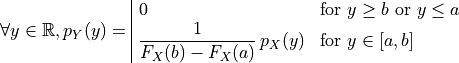# Truncate a distribution¶

In this example we are going to define truncated distributions.

It is possible to truncate a distribution in its lower area, or its upper area or in both lower and upper areas.

In 1-d, assuming a and b bounds, its probability density function is defined as:Is is also possible to truncate a multivariate distribution.

import openturns as ot
import openturns.viewer as viewer
from matplotlib import pylab as plt

ot.Log.Show(ot.Log.NONE)

# the original distribution
distribution = ot.Gumbel(0.45, 0.6)
graph = distribution.drawPDF()
view = viewer.View(graph)truncate on the left

truncated = ot.TruncatedDistribution(distribution, 0.2, ot.TruncatedDistribution.LOWER)
graph = truncated.drawPDF()
view = viewer.View(graph)truncate on the right

truncated = ot.TruncatedDistribution(distribution, 1.5, ot.TruncatedDistribution.UPPER)
graph = truncated.drawPDF()
view = viewer.View(graph)truncated on both bounds

truncated = ot.TruncatedDistribution(distribution, 0.2, 1.5)
graph = truncated.drawPDF()
view = viewer.View(graph)Define a multivariate distribution

dimension = 2
size = 70
sample = ot.Normal(dimension).getSample(size)
ks = ot.KernelSmoothing().build(sample)


Truncate it between (-2;2)^n

bounds = ot.Interval([-2.0] * dimension, [2.0] * dimension)
truncatedKS = ot.Distribution(ot.TruncatedDistribution(ks, bounds))


Draw its PDF

graph = truncatedKS.drawPDF([-2.5] * dimension, [2.5] * dimension,  * dimension)